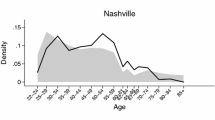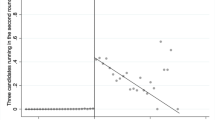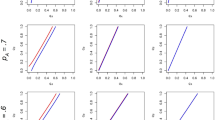# Ties

## Abstract

We tackle the question of the role of pivotality in voter turnout decisions by testing for the first time whether the occurrence of a tied election generates information spillovers onto nearby localities’ subsequent elections. First, we develop a model where voters update their beliefs regarding the probability of their vote being decisive upon observing earlier elections’ outcomes. Next, by exploiting Italian mayoral elections ending in close outcomes during the past two decades and the quasi-experimental conditions created by the staggered electoral calendar, we find a substantial impact on voter turnout rates of exposure to spillovers from tied elections.

This is a preview of subscription content, access via your institution.

### Similar content being viewed by others### Ideology and Vote Choice in U.S. Mayoral Elections: Evidence from Facebook Surveys

Michael W. Sances### Does the number of candidates increase turnout? Causal evidence from two-round elections

Damien Bol & Ria Ivandic### Asymmetric Partisan Voter Turnout Games

Cameron Guage & Feng Fu

1. Congressional Quarterly’s Guide to U S Elections, 6th Edition, 2009, Congressional Quarterly Press, Washington.

2. Of course, how rare an event needs to be in order to get media attention is an empirical issue that we discuss extensively below. As regards Italian local elections, the results of an Internet search for news of close races reported in Sect. 5.2 reveal a disproportionate attention to races ending in exact ties and one-vote-difference results relative to wins by two to five votes of difference.

3. Goeree and Großer (2007) and Taylor and Yildirim (2010a) consider that voters can be informed (similar to the voters in locality 1 in our model) or uninformed (similar to the voters in locality 2) about the true state. However, they assume that the cost of voting is a constant, and the distributions of the political preferences in different states are symmetric for the uninformed voters, which leads to the result that all voters cast their vote with the same probability regardless of type. This is not the case in our model (except when $$\lambda =1/2$$). On the other hand, Taylor and Yildirim (2010b) consider like this paper the situation where the cost of voting is randomly drawn; however, unlike us, though, they assume that all voters are informed about the state regarding the distributions of the political preferences, so there is no uncertainty regarding the state and no room for belief updating.

4. Recall that the probability of abstention by a voter in locality 1 is $$1-\lambda \tau _{1L}^{s*}-(1-\lambda )\tau _{1R}^{s*}$$.

5. The posterior belief for the case of a one-vote-difference outcome can be computed similarly.

6. In our dataset, voter turnout rates are over 75% on average.

7. This pattern emerges in large electorates too. For example, when $$n_{1}=1001$$ and $$\lambda =0.6$$, we find that $${\widehat{\alpha }}\approx 0.0007$$ after a tied event with only 50 votes for each party (with a total turnout rate less than 10%) even when the prior belief is $$\alpha =0.99$$.

8. The sole exception is the possibility (or obligation in some instances) for small-sized municipalities to set up an intermunicipal cooperation agreement or formal institution for the provision of public services that require a minimum scale of production.

9. The average electorate size of the 42 localities that had a tie is about 1600.

10. Section 5 extends the analysis to the elections that are decided by a small number (2-5) of votes.

11. Almost identical results emerge when employing the flexible DiD estimator of de Chaisemartin and D’Haultfoeuille (2022a, 2022b) that applies to binary treatments switching on and off and is robust to heterogeneous and dynamic treatment effects.

12. We should not stress these results too much, though, because, with the exception of the size of the electorate in the short run, the other two controls (number of candidates and win margin) can be suspect of endogeneity or reverse causality.

13. This way, we do not rate as surprises the ties occurring in localities with few hundreds of voters, where a win margin of a handful of votes might exceed 5% of the electorate yet be generally perceived as a pretty close win. 100 votes is the median win margin in the elections preceding ties.

14. None of those variables exhibits any significant pre-trend. The tests of pre-treatment differences in the trajectories of the number of mayors and of the income tax rate between treated and control municipalities have p values of over 0.3 and over 0.9 respectively.

15. The Google news search included the inputs: elezione, sindaco, comune, data, pareggio/differenza di voti (election, mayor, municipality, date, tie/win margin).

16. Interestingly, testing the internal impact of a close election on the next election (as in Sect. 4.1) returns an estimate of a turnout increase that is of the same size as that of a tie, further supporting the hypothesis that the two types of electoral outcomes have roughly the same informational content and have therefore similar consequences within the jurisdiction where they occur, but only the news of the more salient ties succeed in crossing jurisdictional boundaries.

## References

• Agranov M, Goeree J, Romero J, Yariv L (2018) What makes voters turn out: the effects of polls and beliefs. J Eur Econ Assoc 16:825–856

• Baskaran T, Hessami Z (2018) Does the election of a female leader clear the way for more women in politics? Am Econ J Econ Pol 10:95–121

• Börgers T (2004) Costly voting. Am Econ Rev 94:57–66

• Bosch J-C, Eckard E, Singhal V (1998) The competitive impact of air crashes: stock market evidence. J Law Econ 41:503–519

• Burns Z, Chiu A, Wu G (2010) Overweighting of small probabilities. Wiley encyclopedia of operations research and management science

• Bursztyn L, Cantoni D, Funk P, Yuchtman N (2018) Polls, the press, and political participation: the effects of anticipated election closeness. NBER Working Paper No. 23490

• Cancela J, Geys B (2016) Explaining voter turnout: a meta-analysis of national and subnational elections. Elect Stud 42:264–275

• Coate S, Conlin M, Moro A (2008) The performance of pivotal-voter models in small-scale elections: evidence from Texas liquor referenda. J Public Econ 92:582–596

• de Chaisemartin C, D’Haultfoeuille X (2022a) Two-way fixed effects and differences-in-differences with heterogeneous treatment effects: a survey. Econ J (forthcoming)

• de Chaisemartin C, D’Haultfoeuille X (2022b) Difference-in-differences estimators of intertemporal treatment effects. NBER Working Paper No. 29873

• Dhillon A, Peralta S (2002) Economic theories of voter turnout. Econ J 112:332–352

• Duffy J, Tavits M (2008) Beliefs and voting decisions: a test of the pivotal voter model. Am J Polit Sci 52:603–618

• Enos R, Fowler A (2014) Pivotality and turnout: evidence from a field experiment in the aftermath of a tied election. Polit Sci Res Methods 2:309–319

• Faravelli M, Kalayci K, Pimienta C (2020) Costly voting: a large-scale real effort experiment. Exp Econ 23:468–492

• Funk P (2010) Social incentives and voter turnout: evidence from the Swiss mail ballot system. J Eur Econ Assoc 8:1077–1103

• Gelman A, Silver N, Edlin A (2012) What is the probability that your vote will make a difference? Econ Inq 50:321–326

• Gerber A, Green D (2000) The effects of canvassing, telephone calls, and direct mail on voter turnout: a field experiment. Am Polit Sci Rev 94:653–663

• Gerber A, Hoffman M, Morgan J, Raymond C (2020) One in a million: field experiments on perceived closeness of the election and voter turnout. Am Econ J Appl Econ 12:287–325

• Goeree J, Großer J (2007) Welfare reducing polls. Econ Theor 31:51–68

• Großer J, Schram A (2010) Public opinion polls, voter turnout, and welfare: an experimental study. Am J Polit Sci 54:700–717

• Herrera H, Morelli M, Palfrey T (2014) Turnout and power sharing. Econ J 124:F131–F162

• Hyytinen A, Merilainen J, Saarimaa T, Toivanen O, Tukiainen J (2018) When does regression discontinuity design work? Evidence from random election outcomes. Quant Econ 9:1019–1051

• Jarrell G, Peltzman S (1985) The impact of product recalls on the wealth of sellers. J Polit Econ 93:512–536

• Ledyard J (1984) The pure theory of large two-candidate elections. Public Choice 44:7–41

• Levine D, Palfrey T (2007) The paradox of voter participation? A laboratory study. Am Polit Sci Rev 101:143–158

• Lo Prete A, Revelli F (2021) Voter turnout and city performance: evidence from Italian municipalities. J Law Econ Organ 37:168–197

• Lyytikainen T, Tukiainen J (2019) Are voters rational? Eur J Polit Econ 59:230–242

• Mok D, Wellman B, Carrasco J (2010) Does distance matter in the age of the internet? Urban Stud 47:2747–2783

• Morton R, Muller D, Page L, Torgler B (2015) Exit polls, turnout, and bandwagon voting: evidence from a natural experiment. Eur Econ Rev 77:65–81

• Moskowitz D, Schneer B (2019) Reevaluating competition and turnout in U.S. House elections. Q J Polit Sci 14:191–223

• Mulligan C, Hunter C (2003) The empirical frequency of a pivotal vote. Public Choice 116:31–54

• Palfrey T, Rosenthal H (1983) A strategic calculus of voting. Public Choice 41:7–53

• Palfrey T, Rosenthal H (1985) Voter participation and strategic uncertainty. Am Polit Sci Rev 79:62–78

• Riker W, Ordeshook P (1968) A theory of the calculus of voting. Am Polit Sci Rev 62:25–42

• Schachar R, Nalebuff B (1999) Follow the leader: theory and evidence on political participation. Am Econ Rev 89:525–547

• Taylor C, Yildirim H (2010) Public information and electoral bias. Games Econ Behav 68:353–375

• Taylor C, Yildirim H (2010) A unified analysis of rational voting with private values and group-specific costs. Games Econ Behav 70:457–471

• Wakslak C (2012) The where and when of likely and unlikely events. Organ Behav Hum Decis Process 117:150–157

## Acknowledgements

We would like to thank the Editor Elizabeth Maggie Penn, two referees, and seminar participants in Lille (LEM), Lugano (IdEP), Munich (Max Planck Institute), Marseille (AMSE), Taipei (NTU), Torino (SIEP 2019), and particularly Giuseppe Bertola, Pierluigi Conzo, Patricia Funk, Kai Konrad, and Tanguy van Ypersele for useful comments. Yi-fan Chen and Chia-Min Wei provided excellent research assistance. All remaining errors are ours.

## Author information

Authors

### Corresponding author

Correspondence to Tsung-Sheng Tsai.

### Publisher's Note

Springer Nature remains neutral with regard to jurisdictional claims in published maps and institutional affiliations.

## Appendices

### 1.1 Proof of Proposition 1

According to Taylor and Yildirim (2010b), the pivotal probability for an L-type voter is:

\begin{aligned} \begin{array}{lll} &{} &{} \Pi ^s _{1L}(\tau ^s _{1L},\tau ^s _{1R}, \lambda ) \\ &{} &{}\quad = \sum \limits _{k=0}^{\lfloor \frac{n_{1}-1}{2}\rfloor }\left( {\begin{array}{c} n_{1}-1\\ k,k,n_{1}-1-2k\end{array}}\right) (\lambda \tau ^s _{1L})^{k}\left[ (1-\lambda )\tau ^s _{1R} \right] ^{k}\left[ 1-\lambda \tau ^s _{1L}-(1-\lambda )\tau ^s _{1R}\right] ^{n_{1}-1-2k} \\ &{}&{}\qquad + \sum \limits _{k=0}^{\lfloor \frac{n_{1}-1}{2}\rfloor }\left( {\begin{array}{c} n_{1}-1\\ k,k+1,n_{1}-2-2k\end{array}}\right) (\lambda \tau ^s _{1L})^{k}\left[ (1-\lambda )\tau ^s _{1R}\right] ^{k+1}\left[ 1-\lambda \tau ^s _{1L}-(1-\lambda )\tau ^s _{1R} \right] ^{n_{1}-2-2k},\ \ \end{array} \end{aligned}
(A.1)

where $$\lfloor \cdot \rfloor$$ is the integer part of a number. The first term after the equality in (A.1) represents the event where there is a tie, and the second term represents the event where the L-type candidate would lose by one vote without his vote.

Similarly, the pivotal probability for an R-type voter is:

\begin{aligned} \begin{array}{lll} &{} &{} \Pi ^s _{1R}(\tau ^s _{1L},\tau ^s _{1R}, \lambda ) \\ &{} &{}\quad = \sum \limits _{k=0}^{\lfloor \frac{n_{1}-1}{2}\rfloor } \left( {\begin{array}{c}n_{1}-1\\ k,k,n_{1}-1-2k\end{array}}\right) (\lambda \tau ^s _{1L})^{k}\left[ (1-\lambda )\tau ^s _{1R}\right] ^{k}\left[ 1-\lambda \tau ^s _{1L}-(1-\lambda )\tau ^s _{1R} \right] ^{n_{1}-1-2k} \\ &{} &{}\qquad + \sum \limits _{k=0}^{\lfloor \frac{n_{1}-1}{2}\rfloor } \left( {\begin{array}{c}n_{1}-1\\ k,k+1,n_{1}-2-2k\end{array}}\right) (\lambda \tau ^s _{1L})^{k+1}\left[ (1-\lambda )\tau ^s _{1R}\right] ^{k}\left[ 1-\lambda \tau ^s _{1L}-(1-\lambda )\tau ^s _{1R}\right] ^{n_{1}-2-2k}. \end{array} \end{aligned}
(A.2)

By taking the derivatives of the pivotal probabilities in (A.1) and (A.2) with respect to $$\lambda$$, and fixing at the equilibrium $$(\tau ^{s*}_{1L}, \tau ^{s*}_{1R})$$, we have:

\begin{aligned}{} & {} \frac{\partial \Pi ^s_{1L}(\tau ^{s*}_{1L}, \tau ^{s*}_{1R}, \lambda )}{\partial \lambda }\nonumber \\{} & {} \quad =\sum \limits _{k=0}^{\lfloor \frac{n_1-1}{2}\rfloor } \left( {\begin{array}{c}n_1 -1\\ k, k, n_1-2-2k\end{array}}\right) \lambda ^{k-1} (\tau ^{s*}_{1L})^k (1-\lambda )^{k-1} (\tau ^{s*}_{1R})^k \left[ 1-\lambda \tau ^{s*}_{1L}-(1-\lambda )\tau ^{s*}_{1R}\right] ^{n_1-2-2k}\nonumber \\{} & {} \qquad \times \left\{ \frac{k(1-2\lambda )\left[ 1-\lambda \tau ^{s*}_{1L}-(1-\lambda )\tau ^{s*}_{1R}\right] }{(n_1-1-2k)} +\frac{k(1-2\lambda )(1-\lambda )\tau ^{s*}_{1R}}{(k+1)}-\frac{\lambda (1-\lambda )\tau ^{s*}_{1R}}{(k+1)}\right. \nonumber \\{} & {} \qquad + \left. \lambda (1-\lambda )(\tau ^{s*}_{1R}-\tau ^{s*}_{1L}) \left[ 1+\frac{(n_1-2-2k)(1-\lambda )\tau ^{s*}_{1R}}{(k+1)\left[ 1-\lambda \tau ^{s*}_{1L}-(1-\lambda )\tau ^{s*}_{1R}\right] }\right] \right\} ,\quad \textrm{and}\nonumber \\{} & {} \frac{\partial \Pi ^s_{1R}(\tau ^{s*}_{1L}, \tau ^{s*}_{1R}, \lambda )}{\partial \lambda } \end{aligned}
(A.3)
\begin{aligned}{} & {} \quad =\sum \limits _{k=0}^{\lfloor \frac{n_1-1}{2}\rfloor } \left( {\begin{array}{c}n_1-1\\ k, k, n_1-2-2k\end{array}}\right) \lambda ^{k-1} (\tau ^{s*}_{1L})^k (1-\lambda )^{k-1} (\tau ^{s*}_{1R})^k \left[ 1-\lambda \tau ^{s*}_{1L}-(1-\lambda )\tau ^{s*}_{1R}\right] ^{n_1-2-2k}\nonumber \\{} & {} \qquad \times \left\{ \frac{k(1-2\lambda )\left[ 1-\lambda \tau ^{s*}_{1L}-(1-\lambda )\tau ^{s*}_{1R}\right] }{(n_1-1-2k)} +\frac{k(1-2\lambda )\lambda \tau ^{s*}_{1L}}{(k+1)}+\frac{\lambda (1-\lambda )\tau ^{s*}_{1L}}{(k+1)}\right. \nonumber \\{} & {} \qquad + \left. \lambda (1-\lambda )(\tau ^{s*}_{1R}-\tau ^{s*}_{1L}) \left[ 1+\frac{(n_1-2-2k)\lambda \tau ^{s*}_{1L}}{(k+1)\left[ 1-\lambda \tau ^{s*}_{1L}-(1-\lambda )\tau ^{s*}_{1R}\right] }\right] \right\} . \end{aligned}
(A.4)

There are no definite signs in general. We then focus on some special cases to see the effect of $$\lambda$$. Consider a very close election where $$\lambda \rightarrow 1/2$$ in $$s=0$$. In this case, we have $$\tau ^{0*}_{1R}\rightarrow \tau ^{0*}_{1L}$$, according to Lemma 1. Therefore, the terms in the braces in (A.3) and (A.4) approach to zero except the third one. Therefore,

\begin{aligned} \frac{\partial \Pi ^0_{1L}(\tau ^{0*}_{1L}, \tau ^{0*}_{1R}, \lambda )}{\partial \lambda }|_{\lambda \rightarrow 1/2}<0 \ \ \textrm{and} \ \ \frac{\partial \Pi ^0_{1R}(\tau ^{0*}_{1L}, \tau ^{0*}_{1R}, \lambda )}{\partial \lambda }|_{\lambda \rightarrow 1/2}>0. \end{aligned}

Consider a new $$\lambda '$$ which is close to $$\lambda$$ and $$1/2<\lambda ' < \lambda$$. Then under this $$\lambda '$$, since $$\frac{\textrm{d} F}{\textrm{d} c} > 0$$, we know

\begin{aligned} \tau ^{0*}_{1L} < F\left( \frac{1}{2} \Pi ^0_{1L}(\tau ^{0*}_{1L}, \tau ^{0*}_{1R}, \lambda ')\right) \quad \textrm{and} \quad \tau ^{0*}_{1R} > F\left( \frac{1}{2} \Pi ^0_{1R}(\tau ^{0*}_{1L}, \tau ^{0*}_{1R}, \lambda ')\right) . \end{aligned}
(A.5)

Thus, in order to maintain the equality, the new equilibrium $$(\tau ^{0\prime }_{1L}, \tau ^{0\prime }_{1R})$$ under $$\lambda ^\prime$$ must be the case where

\begin{aligned} \tau ^{0\prime }_{1L} > \tau ^{0*}_{1L} \quad \textrm{and}\quad \tau ^{0\prime }_{1R} < \tau ^{0*}_{1R}. \end{aligned}
(A.6)

That is, when a very close election becomes even closer, the pivotal probability for a supporter of the leading (underdog) candidate will be larger (smaller), so that it is more (less) likely for that voter to turn out and vote in the later election.

Another extreme case is $$\lambda \rightarrow 1$$, where the election is dominated by the leading party. Then the terms in the braces in (A.3) and (A.4) approach to zero except the first one. Thus, we have

\begin{aligned} \frac{\partial \Pi ^0_{1L}(\tau ^{0*}_{1L}, \tau ^{0*}_{1R}, \lambda )}{\partial \lambda }|_{\lambda \rightarrow 1}<0 \quad \textrm{and} \quad \frac{\partial \Pi ^0_{1R}(\tau ^{0*}_{1L}, \tau ^{0*}_{1R}, \lambda )}{\partial \lambda }|_{\lambda \rightarrow 1} < 0. \end{aligned}

Consider a new $$\lambda '$$ which is close to $$\lambda$$ and $$\lambda '<\lambda$$. Then under this $$\lambda '$$,

\begin{aligned} \tau ^{0*}_{1L}< F\left( \frac{1}{2} \Pi ^0_{1L}(\tau ^{0*}_{1L}, \tau ^{0*}_{1R}, \lambda ')\right) \quad \textrm{and} \quad \tau ^{0*}_{1R} < F\left( \frac{1}{2} \Pi ^0_{1R}(\tau ^{0*}_{1L}, \tau ^{0*}_{1R}, \lambda ')\right) . \end{aligned}
(A.7)

Thus, in order to maintain the equality, the new equilibrium $$(\tau ^{0\prime }_{1L}, \tau ^{0\prime }_{1R})$$ under $$\lambda '$$ is such that

\begin{aligned} \tau ^{0\prime }_{1L}> \tau ^{0*}_{1L} \quad \textrm{and} \quad \tau ^{0\prime }_{1R} > \tau ^{0*}_{1R}. \end{aligned}
(A.8)

That is, when the election becomes less lopsided, the pivotal probability for a supporter of either party increases, so that it is more likely for a voter to vote. Thus, the total turnout rate increases. $$\square$$

### Appendix B

See Tables 7, 8, 9.

### Appendix C

See Tables 10, 11, 12, 13, 14, 15 and 16.

## Rights and permissions

Springer Nature or its licensor (e.g. a society or other partner) holds exclusive rights to this article under a publishing agreement with the author(s) or other rightsholder(s); author self-archiving of the accepted manuscript version of this article is solely governed by the terms of such publishing agreement and applicable law.

Reprints and Permissions